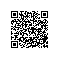PowerShell是一个面向对象的语言，在申明变量的时候不强制要求申明数据类型，使用$开头来申明变量即可。 # 基本数据类型 PowerShell本身是基于.Net开发出来的，所以在.Net中的基本数据类型，在PowerShell中也可以使用，只是在PowerShell中用”[]”来标识具体的数据类型。比如[int],[long],[string],[bool],[double]等。 使用-is来判断某个变量是否指定的数据类型，和C#中的is关键字是一样的。比如：$a=10;
$a -is [int]$a -is [double]

[decimal]$c=10$c.GetType() 可以看到我们的类型为Decimal。

# 数据类型转换

$a=10;$b="10";

$a+$b

$b+$a

[int]$b+$a

($b -as [int])+$a

# List/Array类型

$a=@('a','bb','ccc') 其实不使用@和括号也是可以的，只需要用逗号分割各个Item即可。$a='aaa','bb','c'

$a=@() 与C#不同的是，在PowerShell中，往集合中添加元素，可以使用+=符号。$a=@()
$a+="abc"$a+="dddd"
Write-Host $a 如果要移除某个元素，那么就不简单了，需要使用Where查询（简写为?），找出要保留的元素，然后将保留的元素集合再重新赋值会变量。比如对于字符串集合，我们要移除字符c，那么操作如下：$a=@('aaa','bb','c')
$a$a | ? {$_ -ne 'c'} Write-Host$a

# Hashtable/Dictionary类型

@{Key1=Value1;Key2=Value2;…}

$a=@{Devin="IT";Edward="Finance";Jeneen="Sale"} 使用keys属性可以获得哈希表的Key列表，使用values属性可以获得Value列表。 如果要往哈希表中添加元素，可以使用.Add(Key,Value)方法。比如添加一个员工：$a.Add("Julia","Logisitcs")

if(-not $a.ContainsKey("Julia"))$a.Add("Julia","Logisitcs"
}

$a.Remove("Julia") 如果要访问某个Key对应的值，有两种方法：$a["Devin"
$a.Devin 需要注意的是，直接对哈希表进行Sort-Object是没有效的，我们必须先调用GetEnumerator方法，把哈希表转换后在执行Sort。$a.GetEnumerator() | Sort-Object Name使用钉钉扫一扫加入圈子
+ 订阅